Physics ELECTRIC FIELDS AND ELECTRIC FLUX

### TOPIC COVERED

color{blue}{star} Forces Between Multiple Charges
color{blue}{star} Electric Field
color{blue}{star} Electric Field Lines
color{blue}{star} Electric Flux

### FORCES BETWEEN MULTIPLE CHARGES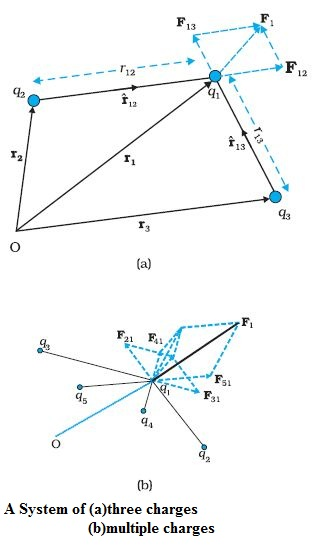color {blue}✍️  Consider a system of three charges  q_1, q_2 and q_3.

The force on charge q_1, due to two other charges q_2, q_3 can be obtained by performing a vector addition of the forces due to each one of these charges. Thus, if the force on q_1 due to q_2 is denoted by vec F_(12),

vec F_(12)=1/(4πε_0)((q_1q_2)/r_(12)^2)hat r_(12)

The force on q_1 due to q_3, denoted by vec F_13, is given by

vec F_(13)=1/(4πε_0)((q_1q_3)/r_(13)^2)hat r_(13)

which is the Coulomb force on q_1 due to q_3

Thus the total forcevec F_1 on q_1 due to the two charges q_2 and q_3 is given as :

vec F_1 = vec F_(12) +vec F_(13) =1/(4πε_0)((q_1q_2)/r_(12)^2)hat r_(12)+1/(4πε_0)((q_1q_3)/r_(13)^2)hat r_(13)

The principle of superposition says that in a system of charges q_1, q_2, ..., q_n, the force on q_1 due to q_2 is the same as given by Coulomb’s law, i.e., it is unaffected by the presence of the other charges q_3, q_4, ..., q_n.

The total force vec F_1 on the charge q_1, due to all other charges, is then given by the vector sum of the forces vec F_(12), vec F_(13), ...,vec F_(1n):

\color{red}ul(★ \color{red} " FORMULA ALERT")

vec F_1 = vec F_(12) +vec F_(13) + ...... +vec F_(1n)

 =1/(4πε_0)[(q_1q_2)/r_(12)^2hat r_(12)+(q_1q_3)/r_(13)^2hat r_(13) +............ +(q_1q_n)/r_(1n)^2hat r_(1n)]

=q_1/(4πε_0)sum_(i=2)^(n)q_i/r_(1i)^2hat r_(1i)

The vector sum is obtained by the parallelogram law of addition of vectors.
Q 3220680511Consider three charges q_1, q_2 , q_3 each equal to q at the vertices of an equilateral triangle of side l. What is the force on a charge Q (with the same sign as q) placed at the centroid of the triangle, as shown in Fig. 1.9?
Class Chapter 1 Example 6Solution:

In the given equilateral triangle ABC of sides of length l, if we draw a perpendicular AD to the side BC, AD = AC cos 30º = ( sqrt(3)//2 ) l and the distance AO of the centroid O from A is (2//3) AD = (1// sqrt(3 )) l. By symmatry AO = BO = CO

Thus,
Force F_1 on Q due to charge q at A = 3/( 4 π ε_0) (Qq)/l^2 along AO

Force  F_2 on Q due to charge q at B = 3/( 4 π ε_0) (Qq)/l^2 along BO

Force F_3 on Q due to charge q at C = 3/( 4 π ε_0) (Qq)/l^2 along CO

The resultant of forces F_2 and F_3 is 3/( 4 π ε_0) (Qq)/l^2 along OA by the parallelogram law. Therefore, the total force on Q = 3/( 4 π ε_0) (Qq)/i^2 (hat r - hat r)

= O, where hat r is the unit vector along OA.
It is clear also by symmetry that the three forces will sum to zero.
Suppose that the resultant force was non-zero but in some direction.
Consider what would happen if the system was rotated through 60º about O.
Q 3230680512Consider the charges q, q, and –q placed at the vertices of an equilateral triangle, as shown in Fig. 1.10. What is the force on each charge?
Class Chapter 1 Example 7Solution:

The forces acting on charge q at A due to charges q at B
and –q at C are F_(12) along BA and F_(13) along AC respectively, as shown
in Fig. 1.10. By the parallelogram law, the total force F_1 on the charge
q at A is given by
F_1 = F hat r_1 where hat r_1 is a unit vector along BC.
The force of attraction or repulsion for each pair of charges has the

same magnitude  F = q^2/(4 pi ε_0 l^2)

The total force F^2 on charge q at B is thus F_2 = F hat r_2, where hat r_2 is a
unit vector along AC.

Similarly the total force on charge –q at C is F_3 = sqrt 3 F hat n , where hat n is
the unit vector along the direction bisecting the ∠BCA.
It is interesting to see that the sum of the forces on the three charges
is zero, i.e.,

F_1 + F_2 + F_3 = 0

The result is not at all surprising. It follows straight from the fact that Coulomb’s law is consistent with Newton’s third law. The proof is left to you as an exercise.

### Electric Field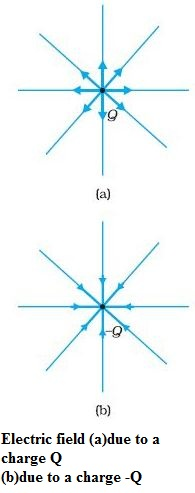Let us consider a point charge Q placed in vacuum, at the origin O.

Another point charge q at a point P, where OP = r, then the charge Q will exert a force on q as per Coulomb’s law.

When another charge q is brought at some point P, the field there acts on it and produces a force. The electric field produced by the charge Q at a point r is given as

vec F(vec r) = 1/(4πε_0)q/(r^2)hat r

where hat r =vec r/r, is a unit vector from the origin to the point r.

\color{blue} ✍️ The above equation specifies the value of the electric field for each value of the position vector r.

color{blue}●The word “field” signifies how some distributed quantity (which could be a scalar or a vector) varies with position.

color{blue}●The effect of the charge has been incorporated in the existence of the electric field. We obtain the force F exerted by a charge Q on a charge q, as

vec F= 1/(4πε_0)(Qq)/r^2 hatr

color{blue}{1.} The charge q also exerts an equal and opposite force on the charge Q .

color{blue}{2.} The electrostatic force between the charges Q and  q can be looked upon as an interaction between charge q and the electric field of  Q and vice versa.

color{blue}{3.} If we denote the position of charge q by the vector  r, it experiences a force F equal to the charge  q  multiplied by the electric field E at the location of q .

vec F(vec r) = q vec E(vec r)

The SI unit of electric field as N/C .

\color{blue} ✍️ Some important remarks made here:

color{blue}{(i)}The electric field due to a charge Q at a point in space may be defined as the force that a unit positive charge would experience if placed at that point.

color{blue}● The charge Q , which is producing the electric field, is called a "source charge".

color{blue}● The charge q, which tests the effect of a source charge, is called a "test charge".

color{blue}●The force F is then negligibly small but the ratio F/q is finite and defines the electric field:

E= lim_(q->0)(F/Q)

color{blue}{(ii)} The ratio F/q  does not depend on q.

color{blue}●The electric field E due to  Q is also dependent on the space coordinate r.

color{blue}●The field exists at every point in three-dimensional space.

color{blue}{(iii)} For a positive charge, the electric field will be directed radially outwards from the charge.

color{blue}{(iv)} For negative, the electric field vector, at each point, points radially inwards.

color{blue}{(v)} The magnitude of the electric field E will also depend only on the distance r .

### Electric Field due to a System of Charges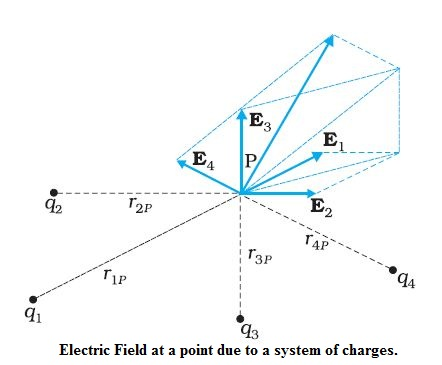Consider a system of charges q_1, q_2, ..., q_n with position vectors r_1, r_2, ..., r_n relative to some origin O. Electric field at a point in space due to the system of charges is defined to be the force experienced by a unit test charge placed at that point, without disturbing the original positions of charges q_1, q_2, ..., q_n.

color{blue}● By using Coulomb’s law and the superposition principle to determine this field at a point P denoted by position vector r.

\color{blue} ✍️Electric field E_1 at r due to q_1 at r_1 is given by

 E_1=1/(4πε_0)q_1/r_(1P)^2 hat r_(1P)

where
hat r _(1P) is a unit vector in the direction from q_1 to P ,
r_(1P) is the distance between q_1 and P .

\color{blue} ✍️Electric field E_2 at r due to q_2 at

 E_2=1/(4πε_0)q_2/r_(2P)^2 hat r_(2P)

where
hat r_(2P) is a unit vector in the direction from q_2 to P,

 r_(2P) is the distance between q_2 and P.

Similar expressions for fields E_3, E_4, ..., E_n due to charges q_3, q_4, ..., q_n.

\color{blue} ✍️By the superposition principle, the electric field E at r due to the system of charges is

E(r) = E_1 (r) + E_2 (r) + … + E_n(r)

=1/(4πε_0)q_1/r_(1P)^2 hat r_(1P)+1/(4πε_0)q_2/r_(2P)^2 hat r_(2P)+......+1/(4πε_0)q_n/r_(nP)^2 hat r_(nP)

E(r)=1/(4πε_0)sum_(i=1)^(n)q_i/r_(iP)^2 hat r_(iP)

E is a vector quantity that varies from one point to another point in space and is determined from the positions of the source charges.

### Physical significance of electric field

The physical significance of the concept of electric field, emerges only when we go beyond electrostatics and deal with time dependent
electromagnetic phenomena.

color{blue}✍️ Suppose we consider the force between two distant charges q_1, q_2 in accelerated motion. Now the greatest speed with which a signal or information can go from one point to another is c, the speed of light.

color{blue}✍️ The accelerated motion of charge q_1 produces electromagnetic waves, which then propagate with the speed c, reach q_2 and cause a force on q_2.

color{blue}✍️ Electric field have an independent dynamics of their own, i.e., they evolve according to laws of their own.

color{blue}✍️ They can also transport energy.
Q 3240680513An electron falls through a distance of 1.5 cm in a uniform electric field of magnitude 2.0 xx 10^4 N C^(–1) [Fig. 1.13(a)]. The direction of the field is reversed keeping its magnitude unchanged and a proton falls through the same distance [Fig. 1.13(b)]. Compute the time of fall in each case. Contrast the situation with that of ‘free fall under gravity’.
Class Chapter 1 Example 8Solution:

In Fig. 1.13(a) the field is upward, so the negatively charged
electron experiences a downward force of magnitude eE where E is
the magnitude of the electric field. The acceleration of the electron is

a_e = eE//m_e

where me is the mass of the electron.

Starting from rest, the time required by the electron to fall through a
distance h is given by  t_e = sqrt( (2h)/a_e) = sqrt ((2h m_e)/(eE))

For e = 1.6 xx 10^(–19) C, m_e = 9.11 xx 10^(–31) kg,

E = 2.0 xx 10^4 N C^(–1) , h = 1.5 xx 10^(–2) m,

t_e = 2.9 xx 10^(–9) s

In Fig. 1.13 (b), the field is downward, and the positively charged
proton experiences a downward force of magnitude eE. The
acceleration of the proton is

a_p = eE//m_p

where m_p is the mass of the proton; m_p = 1.67 xx 10^(–27) kg. The time of
fall for the proton is

 t_p = sqrt( (2h)/a_p) = sqrt ((2h m_p)/(eE)) = 1.3 xx 10^(-7) s

Thus, the heavier particle (proton) takes a greater time to fall through
the same distance. This is in basic contrast to the situation of ‘free
fall under gravity’ where the time of fall is independent of the mass of
the body. Note that in this example we have ignored the acceleration
due to gravity in calculating the time of fall. To see if this is justified,
let us calculate the acceleration of the proton in the given electric
field:

 a_p = (eE)/m_p

 = ( (1 .6 xx 10^(-19) C) xx (2. 0 xx 10^4 N C^(-1) ))/( 1.67 xx 10^(-27) kg)

 = 1.9 xx 10^(12) ms^(-2)

which is enormous compared to the value of g (9.8 m s^(–2) ), the
acceleration due to gravity. The acceleration of the electron is even
greater. Thus, the effect of acceleration due to gravity can be ignored
in this example.
Q 3260680515Two point charges q_1 and q_2, of magnitude +10^(–8) C and
–10^(–8) C, respectively, are placed 0.1 m apart. Calculate the electric
fields at points A, B and C shown in Fig. 1.14.
Class Chapter 1 Example 9Solution:

The electric field vector E_(1A) at A due to the positive charge
q_1 points towards the right and has a magnitude

E_(1A) = ( (9 xx 10^9 N m^2 C^(-2) ) xx (10^(-8) C ))/( 0.05m)^2 = 3.6 xx 10^4 N C^(–1)

The electric field vector E_(2A) at A due to the negative charge q_2 points
towards the right and has the same magnitude. Hence the magnitude
of the total electric field E_A at A is
E_A = E_(1A) + E_(2A) = 7.2 xx 10^4 N C^(–1)

E_A is directed toward the right

The electric field vector E_(1B) at B due to the positive charge q_1 points
towards the left and has a magnitude

 E_(1B) = ( (9 xx 10^9 N m^2 C^(-2) ) xx (10^8 C))/(0.05m)^2 = 3.6 xx 10^4 N C^(–1)

The electric field vector E_(2B) at B due to the negative charge q_2 points
towards the right and has a magnitude

 E_(2B) = ( (9 xx 10^9 Nm^2 C^(-2) ) xx (10^(-8) C))/( 0.05m)^2 = 4 xx 10^3 N C^(–1)

The magnitude of the total electric field at B is

E_B = E_(1B) – E_(2B) = 3.2 xx 10^4 N C^(–1)

E_B is directed towards the left.

The magnitude of each electric field vector at point C, due to charge
q_1 and q_2 is

E_(1C) = E_(2C) = ( (9 xx 10^9 Nm^2 C^(-2) ) xx (10^(-8) C))/( 0.10 m)^2 = 9 xx 10^3 N C^(–1)

The directions in which these two vectors point are indicated in
Fig. 1.14. The resultant of these two vectors is

 E_c = E_1 cos pi/3 + E_2 cos pi/3 = 9 xx 10^3 N C^(–1)

E_c points towards the right.

### ELECTRIC FIELD LINES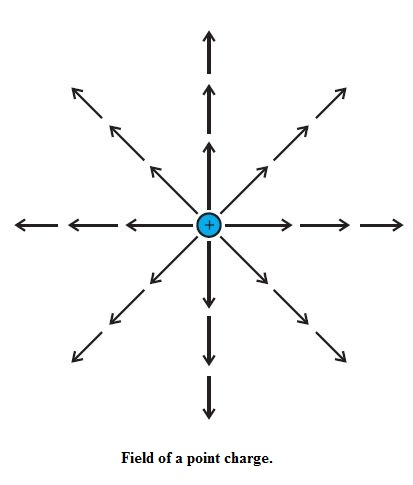color{blue}✍️ E is strong near the charge, so the density of field lines is more near the charge and the lines are closer. Away from the charge, the field gets weaker and the density of field lines is less, resulting in well-separated lines.color{blue}✍️ The field lines of a single positive charge are radially outward while those of a single negative charge are radially inward. The field lines around a system of two positive charges (q, q) give a vivid pictorial description of their mutual repulsion, while those around the configuration of two equal and opposite charges (q,–q), a dipole, show clearly the mutual attraction between the charges.

color{blue}✍️ The field lines follow some important general properties:color{blue}{ (i)} Field lines start from positive charges and end at negative charges. If there is a single charge, they may start or end at infinity.

color{blue}{(ii)} In a charge-free region, electric field lines can be taken to be continuous curves without any breaks.

color{blue}{(iii)} Two field lines can never cross each other.

color{blue}{(iv)} Electrostatic field lines do not form any closed loops.

### ELECTRIC FLUX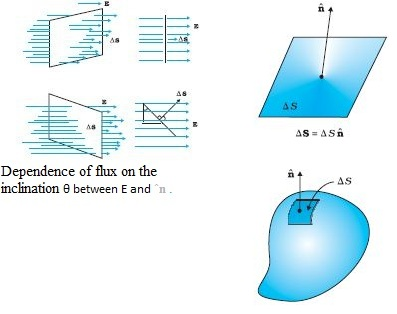Electric flux Δφ through an area element ΔS is defined by:

Δφ = E.ΔS = E ΔS cosθ

which, is proportional to

color{blue}{1.} The number of field lines cutting the area element.

color{blue}{2.} The angle θ here is the angle between E and ΔS.

\color{blue} ✍️ θ is the angle between E and the outward normal to the area element.

The unit of electric flux is N C^(–1) m^2.

To calculate the total flux through any given surface. All we have to do is to divide the surface into small area elements, calculate the
flux at each element and add them up. Thus, the total flux φ through a surface S is

φ ~ Σ E.ΔS

The approximation sign is put because the electric field E is taken to be constant over the small area element. This is mathematically exact only when you take the limit ΔS → 0 and the sum is written as an integral.